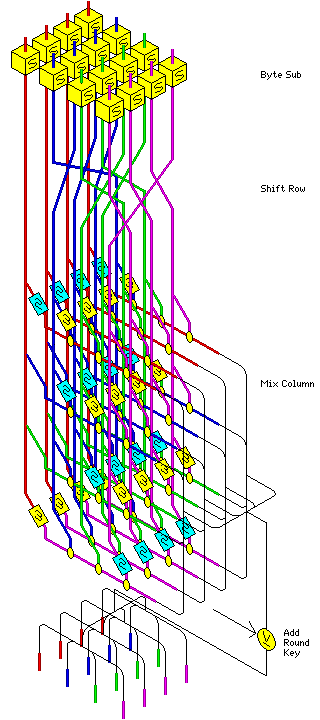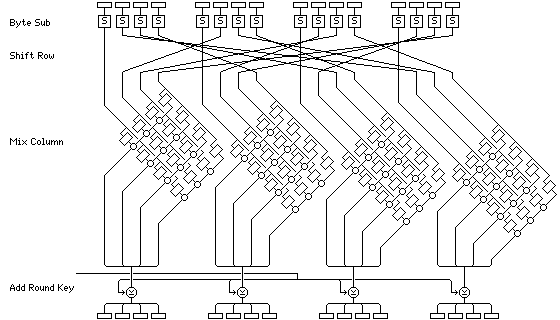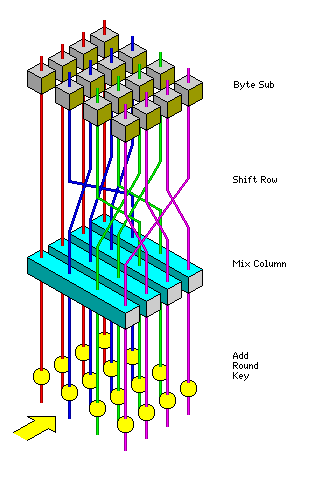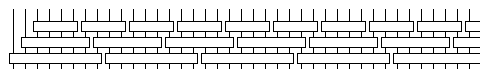[Next] [Up/Previous] [Index]

# The Advanced Encryption Standard (Rijndael)

The block cipher Rijndael is designed to use only simple whole-byte operations. Also, it provides extra flexibility over that required of an AES candidate, in that both the key size and the block size may be chosen to be any of 128, 192, or 256 bits. (During an early stage of the AES process, a draft version of the requirements would have required each algorithm to have three versions, with both the key and block sizes equal to each of 128, 192, and 256 bits. This was later changed to make the three required versions have those three key sizes, but only a block size of 128 bits, which is more easily accomodated by many types of block cipher design.)

The original description of Rijndael is available at: http://www.esat.kuleuven.ac.be/~rijmen/rijndael/.However, the variations of Rijndael which act on larger block sizes apparently will not be included in the actual standard, on the basis that the cryptanalytic study of Rijndael during the standards process primarily focused on the version with the 128-bit block size.

Rijndael is a relatively simple cipher in many respects.

Rijndael has a variable number of rounds. Not counting an extra round performed at the end of encipherment with one step omitted, the number of rounds in Rijndael is:

• 9 if both the block and the key are 128 bits long.
• 11 if either the block or the key is 192 bits long, and neither of them is longer than that.
• 13 if either the block or the key is 256 bits long.

To encipher a block of data in Rijndael, you first perform an Add Round Key step (XORing a subkey with the block) by itself, the regular rounds noted above, and as already noted, the final round with the Mix Column step, as described below, omitted.

### The Rounds

Each regular round involves four steps. First is the Byte Sub step, where each byte of the block is replaced by its substitute in an S-box.

The specification for Rijndael only provided an explanation of how the S-box was calculated: the first step was to replace each byte with its reciprocal in the same GF(2^8) as used below in the Mix Column step, except that 0, which has no reciprocal, is replaced by itself (since it isn't anything's reciprocal either, it is the only value not used, so that makes sense) then a bitwise modulo-two matrix multiply was used, and finally the hexadecimal number 63 is XORed with the result. (Not C6, x7 is the MSB, if the diagram in the specification appears confusing.)

The S-box is:

``` 99 124 119 123 242 107 111 197
48   1 103  43 254 215 171 118
202 130 201 125 250  89  71 240
173 212 162 175 156 164 114 192
183 253 147  38  54  63 247 204
52 165 229 241 113 216  49  21
4 199  35 195  24 150   5 154
7  18 128 226 235  39 178 117
9 131  44  26  27 110  90 160
82  59 214 179  41 227  47 132
83 209   0 237  32 252 177  91
106 203 190  57  74  76  88 207
208 239 170 251  67  77  51 133
69 249   2 127  80  60 159 168
81 163  64 143 146 157  56 245
188 182 218  33  16 255 243 210
205  12  19 236  95 151  68  23
196 167 126  61 100  93  25 115
96 129  79 220  34  42 144 136
70 238 184  20 222  94  11 219
224  50  58  10  73   6  36  92
194 211 172  98 145 149 228 121
231 200  55 109 141 213  78 169
108  86 244 234 101 122 174   8
186 120  37  46  28 166 180 198
232 221 116  31  75 189 139 138
112  62 181 102  72   3 246  14
97  53  87 185 134 193  29 158
225 248 152  17 105 217 142 148
155  30 135 233 206  85  40 223
140 161 137  13 191 230  66 104
65 153  45  15 176  84 187  22
```

Note that 63 in hexadecimal is 3 plus 6*16, or 36+60 or 96, and that is 99, as begins the table.

Next is the Shift Row step. Considering the block to be made up of bytes 1 to 16, these bytes are arranged in a rectangle, and shifted as follows:

```from            to
1  5  9 13      1  5  9 13
2  6 10 14      6 10 14  2
3  7 11 15     11 15  3  7
4  8 12 16     16  4  8 12
```

Blocks that are 192 and 256 bits long are shifted like this:

```from                  to
1  5  9 13 17 21      1  5  9 13 17 21
2  6 10 14 18 22      6 10 14 18 22  2
3  7 11 15 19 23     11 15 19 23  3  7
4  8 12 16 20 24     16 20 24  4  8 12

and
from                        to
1  5  9 13 17 21 25 29      1  5  9 13 17 21 25 29
2  6 10 14 18 22 26 30      6 10 14 18 22 26 30  2
3  7 11 15 19 23 27 31     15 19 23 27 31  3  7 11
4  8 12 16 20 24 28 32     20 24 28 32  4  8 12 16
```

Note that in the 256 bit case, the rows are shifted 1, 3, and 4 places to the left, instead of 1, 2, and 3 places as for the other two block sizes.

Next comes the Mix Column step. Matrix multiplication is performed: each column, in the arrangement we have seen above, is multiplied by the matrix:

``` 2 3 1 1
1 2 3 1
1 1 2 3
3 1 1 2
```

However, this multiplication is done over GF(2^8). This means that the bytes being multiplied are treated as polynomials rather than numbers. Thus, a byte "muliplied" by 3 is that byte XORed with that byte shifted one bit left.

If the result has more than 8 bits, the extra bits are not simply discarded: instead, they're cancelled out by XORing the binary 9-bit string 100011011 with the result (shifted right if necessary). This string stands for the generating polynomial of the particular version of GF(2^8) used; a similar technique is used in cyclic redundancy checks.

For example, multiplying the binary string 11001010 by 3 within this Galois Field works like this:

```        11001010
*       11
---------
11001010
11001010
---------
100011011  (this is XORed, instead of subtracting 256)
---------
1000101
```

The final step is Add Round Key. This simply XORs in the subkey for the current round.

Although a three-dimensional color diagram of the round has already appeared at the beginning of the page, the Rijndael round can also be illustrated in a more conventional manner:The extra final round omits the Mix Column step, but is otherwise the same as a regular round.

Thus, the sequence of steps in Rijndael is:

```ARK

BSB
SR
MC
ARK

BSB
SR
MC
ARK

...

BSB
SR
MC
ARK

BSB
SR
ARK
```

Because it begins and ends with an ARK (Add Round Key) step, there is no wasted unkeyed step at the beginning or end. The sequence of operations is important for facilitating decipherment, as well.

Although the sequence is not symmetrical, the order of some of the steps in Rijndael could be changed without affecting the cipher. The Byte Sub step could just as easily be done after the Shift Row step as before it.

That would change

```A BSMA BSMA ... BSMA BSA
```

to

```A SBMA SBMA ... SBMA SBA
```

If, on the other hand, we reversed the original sequence, we would get

```ASB AMSB ... AMSB AMSB A
```

Although these look similar, except for the different positions of the spaces, which just mark what are termed "rounds" in the algorithm, wherever the sequence "MA" appears in the modified, but equivalent, algorithm, the sequence "AM" appears in the reverse sequence, required for decryption.

Of course, the steps also need to be reversed as well: ARK is an XOR, so that is its own inverse, but the matrix in the Mix Column step needs to be replaced with its inverse, and the S-box in the Byte Sub step needs to be replaced with its inverse for decryption as well.

What about the switch between "MA" and "AM", though? Do we need to change the order of operations for decryption?

No; with matrix multiplication, the distributive property also applies, so the round keys involved in such a reversal merely need to be multiplied by the (inverse) Mix Column matrix, and then they can be XORed in at the same time (of course, in reverse order) as was used for encryption. (XOR corresponds to addition in GF(2^8), which is where the multiplications are performed.)

The matrix for the inverse Mix Column step is:

```14 11 13  9
9 14 11 13
13  9 14 11
11 13  9 14
```

as can quickly be verified by making use of its symmetry, and once the answer is known:

```1110 1011 1101 1001  01 00 00 00
1001 1110 1011 1101  00 01 00 00
1101 1001 1110 1011  00 00 01 00
1011 1101 1001 1110  00 00 00 01

111  101  110  100  01 01 00 00
110  100  111  101  00 00 01 01
1100 1000 1110 1010  00 00 10 10
1011 1101 1000 1110  01 01 10 10
0    0    1    0  01 01 10 11
```

Since we are now performing Galois Field multiplication by larger numbers, another example of Galois Field multiplication may be useful here. To multiply the binary string 10100011 by 14 within this Galois Field will work like this:

```        10100011
*     1110
-----------
10100011
10100011
10100011
-----------
100011011    (this is XORed, instead of subtracting 256)
-----------
1010111110
100011011   (this is XORed, instead of subtracting 256)
----------
10001000
```

This example illustrates how one repeatedly XORs the value 100011011, shifted left as many times as necessary to clear the first bit of the result, in order to obtain a final result which fits within eight bits.

### The Key Schedule

For keys 128 and 192 bits in length, the subkey material, which consists of all the round keys in order, consists of the original key, followed by stretches, each the length of the original key, consisting of four-byte words such that each word is the XOR of the preceding four-byte word and either the corresponding word in the previous stretch or a function of it. For the first word in a stretch, the word is first rotated one byte to the left, and then its bytes are transformed using the S-box from the Byte Sub step, and then a round-dependent constant is XORed to its first byte.

The round constants are:

```  1   2   4   8  16  32  64 128
27  54 108 216 171  77 154  47
94 188  99 198 151  53 106 212
179 125 250 239 197 145  57 114
228 211 189  97...
```

which are, in binary:

```00000001 00000010 00000100 00001000 00010000 00100000 01000000 10000000
00011011 00110110 01101100 11011000 10101011 01001101 10011010 00101111
01011110 10111100 01100011 11000110 10010111 00110101 01101010 11010100
10110011 01111101 11111010 11101111 11000101 10010001 00111001 01110010
11100100 11010011 10111101 01100001...
```

the successive powers of 2 in the representation of GF(2^8) used. Successive powers of 3, unlike 2, include all the values from 1 to 255, and thus several implementations of Rijndael use tables of the powers of 3, and the inverse table giving the discrete logarithm in that field, to facilitate calculations, but the round constants are still the powers of 2. (I'm not entirely sure why one needs log and antilog tables when all one is doing is muliplying by 2 and by 3, but doubtless there is a nonobvious way to implement Rijndael with greater efficiency that makes use of them. The inverse of the matrix used in the Mix Column step has the values 9, 11, 13, and 14 as its coefficients, however, so this could simply serve to limit the number of tables needed for both enciphering and deciphering, replacing six tables by two.)

For keys 256 bits in length, in addition, the S-box from the Byte Sub step alone is applied to the word from the preceding stretch for the fifth word in a stretch in addition.

### Attacks on RijndaelAlthough this page (and the preceding one) already have several diagrams of Rijndael, I've included yet another one, one even more simplified than the one appearing on the previous page, to make it easier to "see the forest for the trees", and see both that the Mix Column step indeed only mixes columns, and is the only place where that happens, and how the Shift Row step works with the Mix Column step to ensure that all parts of the block impinge on each other.

The standard techniques of differential and linear cryptanalysis can be adapted to be used against Rijndael. Because of the way matrix multiplication works, and because in GF(2^8), all the coefficients of the Mix Column matrix (as indeed all numbers from 1 to 255) have reciprocals, a specific attack, originally developed for use against its predecessor Square, called the "Square attack", can be used as well.

If one uses 256 blocks of chosen plaintext, where every byte but one is held constant, and that one is given all 256 possible values, then after one round of Rijndael, four bytes will go through all 256 possible values, and the rest of the bytes will remain constant. After a second round, sixteen bytes will each go through all 256 possible values, without a single duplicate, in the encipherment of the 256 blocks of chosen plaintext. (For a 128-bit block, this is every byte; for larger blocks, the rest of the bytes will remain constant.) This interesting property, although not trivial to exploit, can be used to impose certain conditions on the key when one additional round, before or after the two rounds involved, is present.

The possibility of this attack was first noted by the developers of Square and Rijndael themselves, and was noted in the paper that initially described Square.

The availability of different block sizes with Rijndael permits a cute variant of the "bricklaying" mode proposed for Triple-DES to be used with it:Here, each vertical line represents 32 bits, and the three layers use the 128, 192, and 256 bit block sizes in order.

Despite the fact that Rijndael has a very different structure from that of DES, and in some ways could be said to more closely resemble SAFER, because the Byte Sub step directly alters the bytes to be encrypted, and the Mix Column step causes every byte in a column to affect every other byte there, somewhat as the PHT stage in SAFER involves the whole block, it is still possible to relate the fundamental steps in Rijndael to parts of DES based on the function they perform in contributing to the step of the overall cipher.

The Add Round Key step clearly corresponds to the XOR of subkey material with the input to the f-function.

The Mix Column step is where the different bytes interact with each other, so it corresponds to the XOR of the f-function output with the left half of the block in DES.

The Byte Sub step contributes the nonlinearity in Rijndael, and so it corresponds to the f-function itself.

The Shift Row step ensures that the different bytes of each row do not only interact with the corresponding byte in other rows. Thus, it corresponds to permutation P within DES.

In drawing an analogy between Rijndael and DES, it is very easy, based on a superficial glance at Rijndael, and the appearance of the Shift Row step, to view it as corresponding to the swapping of halves of the block within DES. In fact, Rijndael does not require a step with this function, because the Mix Column step in Rijndael causes every byte in a column to alter every other byte in the column, so no step is needed that swaps rows.

This subtle point is actually quite important. The number of regular rounds in Rijndael is always an odd number; without a Mix Column step, the last round involves no interaction between bytes, so it makes sense not to count it as a "real" round. Thus, it is not a multiple of the number of columns, which is 4, 6, or 8, depending on the size of the block.

Differential cryptanalysis attacks on DES become quite a bit easier for variants of DES with an odd number of rounds. Thus, if the Shift Row step in Rijndael had corresponded to swapping halves of the block, this would have possibly been an important weakness in Rijndael. It may still be a slight flaw, but existing cryptanalytic results against Rijndael do not seem to exhibit a pattern indicating this.

The key schedule for Rijndael can be carried out equally well with keys that are 160 or 224 bits long, since it only requires that the key size be a multiple of 32 bits. Even block sizes of 160 and 224 bits are possible as well. A revised version of the Rijndael specification includes these as extensions, and the rule for the number of rounds to use is based on the larger of the block size and the key size, with 10 regular rounds (11 rounds in total) corresponding to 160 bits, and 12 regular rounds (13 rounds in total) corresponding to 224 bits.

Thus, one can obtain 12 regular rounds, which I view as desirable for the 128-bit and 192-bit block sizes, by specifying a key of 224 bits. As a nice bonus, the number of 32-bit words in 224 bits is seven, which is relatively prime to either four or six, which appears, at least from a naïve point of view, as if it might improve the key schedule at least slightly as well.

This chart, showing the number of rounds for different block sizes and key sizes, also indicates when these apparently desirable properties are obtained:

```* : number of regular rounds is a multiple of the
number of 32-bit words in a block
+ : number of 32-bit words in the key is relatively
prime to the number of 32-bit words in a block

Key   Block
128 160 192 224 256
128    10  11  12  13  14   10 is a multiple of 5
+ *     +          4 is relatively prime to 5 and 7

160    11  11  12  13  14   10 is a multiple of 5
+     * +   +   +      5 is relatively prime to 4, 6, 7, and 8

192    12  12  12  13  14
+       +          6 is relatively prime to 5 and 7

224    13  13  13  13  14   12 is a multiple of 4 and 6
+ * +   + *     +      7 is relatively prime to 4, 5, 6, and 8

256    14  14  14  14  14
+       +          8 is relatively prime to 5 and 7

288    15  15  15  15  15   14 is a multiple of 7
+   +       + * +      9 is relatively prime to 4, 5, 7, and 8

320    16  16  16  16  16   15 is a multiple of 5
*     +         10 is relatively prime to 7

352    17  17  17  17  17   16 is a multiple of 4 and 8
+ * +   +   +   + *   11 is relatively prime to 4, 5, 6, 7, and 8
```

Thus, there is at least one "ideal" key size for every possible block size:

```Block
size    Ideal key sizes
128    224  352
160    128
192    224
224    288
256    352
```

once again, I reiterate, this is only significant if these essentially minor concerns, as seen from a naïve point of view, have any validity.

Incidentally, for a block size of 224 bits, the Shift Row step is again altered, but not in quite the same way as for a 256-bit block. Only the last row, not the last two rows, is shifted by an extra byte position. Also, the additional Byte Sub step noted for 256-bit keys is added to the key schedule for all key sizes greater than 192 bits, so it applies to the 224-bit key as well as to all key sizes greater than 256 bits.

That the design of the S-box involves the same GF(2^8) as the Mix Column step might also appear to be a concern. However, the Rijndael S-box is nearly ideal in resistance to differential cryptanalysis, and is also excellent in avoiding any approximations in GF(2^8) usable in the GF(2^8) equivalent of linear cryptanalysis.

The choice of Rijndael over the other finalist algorithms, also believed to be highly secure, was based primarily on its efficiency and low memory requirements; this, coupled with the fact that existing cryptanalyses of Rijndael are based on reduced-round variants somewhat close to the actual cipher (although the results close to the actual number of rounds are quite impractical to exploit) means that some controversy, even if not the intense controversy surrounding DES, may haunt the new AES as well.

Rijndael was preceded by the block cipher Square, which was somewhat similar. However, instead of a Shift Row step, a transpose of the square matrix of bytes was used; in effect, this meant that diffusion over the whole block was obtained by alternating Mix Column steps with Mix Row steps. Specific attacks were found against Square, against which Rijndael was strengthened.

Finally, I will propose a minor variation to Rijndael that might make cryptanalysis more difficult.

Instead of subjecting all the columns of the block to the same operation in the Mix Column step, every second column, instead of being subjected to a matrix multiplication, could instead be subjected to a scaled-down version of the PHT stage in SAFER. Since only four bytes are involved, this would mean two stages of PHT operations, and the bytes in the second and third rows would be swapped between the stages. To avoid major changes to the shift row step, the pattern of operations should be MPMP for two rounds, and then PMPM for two rounds; also, the bytes in the second and third rows should be swapped back after the second PHT stage (or the PHT operations would be performed "in place", which is equivalent). By using two completely unrelated operations, but both of which completely mix all the bytes in a column, resistance to analysis should be improved.

However, although this is a different form of mixing, and will thus block the Square attack, it is also a weaker form of mixing, and thus would need to be combined with an increase in the number of rounds.

[Next] [Up/Previous] [Index]